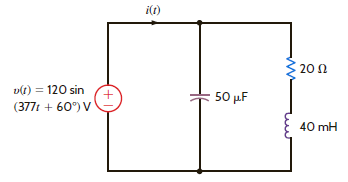Problem

# Find the current i(t) in the network in Fig. E8.8.Figure E8.8.

Find the current i(t) in the network in Fig. E8.8.Figure E8.8.

#### Step-by-Step Solution

Solution 1

Refer to Figure E8.8 in the text book.

The impedances of the individual elements are as follows:

$$Z_{R}=20 \Omega$$

From the voltage source, the angular frequency is 377 .

Write the expression for the frequency.

$$f=\frac{\omega}{2 \pi}$$

Calculate the frequency by substituting 377 for $$\omega$$.

\begin{aligned} f &=\frac{\omega}{2 \pi} \\ &=\frac{377}{2 \pi} \\ &=60 \mathrm{~Hz} \end{aligned}

The impedance of the inductance is as follows:

$$Z_{L}=j \omega L$$

Calculate the inductance impedance by substituting $$60 \mathrm{~Hz}$$ for $$f$$ and $$40 \mathrm{mH}$$ for $$L$$.

\begin{aligned} Z_{L} &=j \omega L \\ &=j(2 \pi)(60)\left(40 \times 10^{-3}\right) \\ &=j 15.07 \Omega \end{aligned}

The impedance of the capacitance is as follows:

$$Z_{C}=\frac{-j}{\omega C}$$

Calculate the capacitance impedance by substituting $$60 \mathrm{~Hz}$$ for $$f$$ and $$50 \mu \mathrm{H}$$ for $$C$$.

\begin{aligned} Z_{C} &=\frac{-j}{\omega C} \\ &=\frac{-j}{2 \pi(60)\left(50 \times 10^{-6}\right)} \\ &=-j 53.07 \Omega \end{aligned}

Write the expression for the equivalent impedance.

$$Z=\left(Z_{R}+Z_{L}\right) \| Z_{C}$$

Calculate the equivalent impedance.

Substitute $$20 \Omega$$ for $$Z_{R}, j 15.07 \Omega$$ for $$Z_{L}$$ and $$-j 53.07 \Omega$$ for $$Z_{C}$$ in the equation.

\begin{aligned} Z &=\left(Z_{R}+Z_{L}\right) \| Z_{C} \\ &=(20+j 15.07) \|(-j 53.07) \\ &=\frac{799.76-j 1061.4}{20-j 38} \\ &=\frac{1328.97 \angle-53^{\circ}}{42.94 \angle-62.24^{\circ}} \\ &=30.94 \angle 9.24^{\circ} \Omega \end{aligned}

The voltage source is as follows:

\begin{aligned} v(t) &=120 \sin \left(377 t+60^{\circ}\right) \mathrm{V} \\ &=120 \cos \left(377 t+60^{\circ}-90^{\circ}\right) \mathrm{V} \\ &=120 \cos \left(377 t-30^{\circ}\right) \mathrm{V} \\ &=120 \angle-30^{\circ} \mathrm{V} \end{aligned}

Write the expression for the current in the circuit.

$$\mathrm{I}=\frac{\mathrm{V}}{\mathrm{Z}}$$

Calculate the current in the circuit.

Substitute $$120 \angle-30^{\circ}$$ for $$\mathrm{V}$$ and $$30.94 \angle 9.24^{\circ}$$ for $$\mathrm{Z}$$ in the equation.

\begin{aligned} I &=\frac{V}{Z} \\ &=\frac{120 \angle-30^{\circ}}{30.94 \angle 9.2^{\circ}} \\ &=3.88 \angle-39.2 \mathrm{~A} \end{aligned}

Convert the current in phasor form to time domain.

$$i(t)=3.88 \cos \left(377 t-39.2^{\circ}\right) \mathrm{A}$$

Therefore, the current in the circuit is $$3.88 \cos \left(377 t-39.2^{\circ}\right) \mathrm{A}$$.